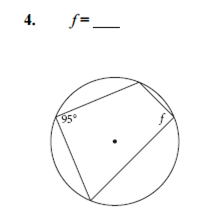# 9.3 Arcs and Angles 2

## Investigation 2: Cyclic Quadrilaterals Now you’ll apply your previous discoveries to the angles of a quadrilateral inscribed in a circle, which is called a cyclic quadrilateral.

Investigate 1. Look for relationships between pairs of angles in the quadrilateral. Use a calculator to check any relationships you discover, then write a conjecture (Cyclic Quadrilateral Conjecture). 2. Explain why the Cyclic Quadrilateral Conjecture is true. (What kinds of angles did you measure? What is the sum of the arc measures of the two arcs intercepted by opposite angles in the quadrilateral?)

Check all that applyCheck all that apply